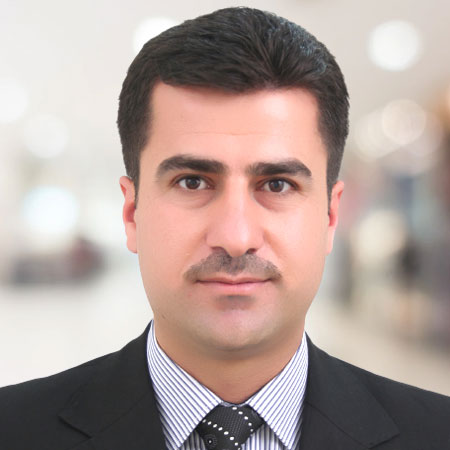Professor
University of Sulaimani, Iraq

Highest Degree
Ph.D. in Applied Mathematics from University of Sulaimani, Iraq

# Biography

Dr. Faraidun Kadir Hamasalh is currently working as Professor at Sulaimani University, Iraq. He has completed his Ph.D. in Mathematics from same University. His field of specialization includes Numerical Analysis, Interpolation by Spline function, and Fluid Flow. He is member of many committees of Sulaimani University. He also attended number of workshops, conferences and training.

# Area of Interest:

Mathematics
100%
Numerical Analysis
62%
Approximation Theory
90%
Fluid Mechanics
75%
Applied Mathematics
55%

Books
5
Chapters
5
Articles
65
Abstracts
0

# Selected Publications

1. Mohammed, P.O., F.K. Hamasalh and T. Abdeljawad, 2021. Difference monotonicity analysis on discrete fractional operators with discrete generalized mittag-leffler kernels. Adv. Differ. Equations, Vol. 213. 10.1186/s13662-021-03372-2.
2. Hafez, Y. and A. Labban, 2020. A recent study of the relationship between the precipitation rate over Saudi Arabia in the fall and climatic indices. Adv. Meteorol., 2020: 1-11.
3. Mohammed, P. and F. Hamasalh, 2019. New conformable fractional integral inequalities of hermite-hadamard type for convex functions. Symmetry, 10.3390/sym11020263.
4. Jwamer, F., K. Hama, F.K. Hamasalh, K. Muhhamad, R. Ghafoor and R.R. Qadir, 2017. Estimates of error bounds for seventic spline polynomial. NED Univ. J. Res., 15: 1-10.
5. Hamasalh, F.K. and P.O. Muhammed, 2017. Computational non-polynomial spline function for solving fractional Bagely-Torvik equation. Math. Sci. Lett., 6: 83-87.
6. Mohammed, P.O. and F.K. Hamasalh, 2016. Twelfth degree spline with application to quadrature. SpringerPlus, Vol. 5. 10.1186/s40064-016-3711-2.
CrossRef  |  ASCI  |
7. Mohammed, P.O. and F.K. Hamasalh, 2016. Twelfth degree spline with application to quadrature. Springer Plus, 10.1186/s40064-016-3711-2.
8. Jwamer, K.H.F. and F.K. Hamasalh, 2016. Best error bounds for splines of degree seven. ZANCO J. Pure Applied Sci., 28: 33-40.
9. Hamasalh, F.K., S.M. Rasheed and P.O. Muhammad, 2016. Composition Fractional Integral Inequality for the Reiman-Liouville type with applications. J. Zankoy Sulaimani-A, 18: 227-230.
10. Hamasalh, F.K. and P.O. Muhammed, 2016. Numerical treatment of lacunary spline function of fractional order. J. Inform. Comput. Sci., 11: 163-168.
11. Hamasalh, F.K. and P.O. Muhammed, 2016. Computational Method for Fractional Differential Equations Using Nonpolynomial Fractional Spline. Math. Sci. Lett., 5: 131-136.
12. Faraidun, K.H., K.F.H. Jwamer and S.A. Mohammed, 2016. An algorithm for computing spline function. J. Zankoy Sulaimani, 18: 962-972.
13. Saeed, R.K., K.H.E. Jwamer and F.K. Hamasalh, 2015. Introduction to Numerical Analysis. 3rd Edn., Sulaimani University, Kurdistan Region, Iraq.
14. Hamasalh, F.K., 2015. Fractional polynomial spline for solving differential equations of fractional order. Math. Sci. Lett., 4: 291-296.
15. Hamasalh, F.K. and P.O. Muhammad, 2015. Numerical Solution of Fractional Differential Equations by using Fractional Spline Model. J. Inf. Comput. Sci., 10: 098-105.
16. Hamasalh, F.K. and P.O. Muhammad, 2015. Generalized quartic fractional spline interpolation with applications. Int. J. Open Prob. Comput. Math., 8: 67-80.
17. Hamasalh, F.K. and P.O. Muhammad, 2015. Generalized nonpolynomial spline method by fractional order. Gen. Math. Notes, 28: 42-53.
18. Hamasalh, F.K. and P.O. Muhammad, 2015. Analysis of fractional splines interpolation and optimal error bounds. Am. J. Numer. Anal., 3: 30-35.
19. Hamasalh, F.K., 2014. Approximate solution of initial value problems by means of ninth degree spline. J. Zankoy Sulaimani-Part A, Vol. 16. .
20. Hamasalh, F.K. and S.A. Tahir, 2014. Unique lacunary interpolations with estimate errors bound. Am. J. Appl. Math. Stat., 2: 88-91.
21. Manna, S.A., F.K. Hamasalh and H.N. Aziz, 2013. An approximate solution of some differential equations with new type of interpolation. Asian J. Math. Stat., 6: 33-42.
22. Jwamer, K.H.F. and F.K. Hamasalh, 2013. Inhomogeneous lacunary interpolation and optimization errors bound of seventh spline. Am. J. Applied Math. Stat., 1: 46-51.
23. Hamasalh, F.K., G. Joseph and G. Najmadeen, 2013. Spline computation for solving magnetohidrodynamics free convection flow. Int. J. Comput. Technol., 5: 158-166.
24. Hama-Salh, F.K. and D.M. Salih, 2013. Minimize of error estimation and approximate solution of fifth order initial value problems. J. Zankoy Sulaimani-Part A, 15: 117-124.
25. Saeed, R.K., F.K. Hamasalh and G.W. Sadiq, 2012. Convergence of ninth spline function to the solution of a system of initial value problems. World Appl. Sci. J., 16: 1360-1367.
26. Jwamer, K.H.F., H.S.F. Kadir and A.J. Muhamad, 2012. Estimation of pit excavation volume by fifth degree polynomial. Int. J. Modern Eng. Res., 2: 1511-1517.
27. Jwamer, K.H. and F.K. Hamasalh, 2012. On optimality of lacunary interpolation for recovery of C6 seventh degree spline. Int. J. Open Problems Comput. Math., 5: 103-113.
28. Hamasalh, F.K., 2012. Applied lacunary interpolation for solving boundary value problems. Int. J. Mod. Eng. Res., 2: 118-123.
29. Karwan, H.F., K. Faraidun and K.G. Radha, 2011. Approximation new error bounded by spline degree six. Acta Universitatis Apulensis, 2015: 201-210.
30. Hamasalh, F.K., A.A. Abdulla and K.M. Qadir, 2011. Computation of fifth degree of spline function model by using C++ programming. Int. J. Comput. Sci., 8: 289-294.
31. Faraj, J.K.H., H.S.F. Kadir and A.J. Muhamad, 2011. Irregular boundary area computation by quantic hermite polynomial. Int. J. Contemp. Math. Sci., 6: 123-132.
32. Hamasalh, F.K., A. Al-Bayati and R. Saeed, 2010. Investigation in Lacunary Interpolations with Applications. Lambert Academic Publishing, Germany, ISBN: 978-3-8465-1153-4.
33. Hamasalh, F.K., 2010. Inhomogeneous lacunary interpolation by splines (0,2,0,1,4) Case. Asian J. Math. Stat., 3: 211-224.
34. Hamasalh, F.K., 2010. Numerical solution for fifth order initial value problems using lacunary interpolation. J. Duhok Univ., 13: 128-134.
35. Hamasalh, F.K. and K.H.F. Jwamer, 2010. Cauchy problem and modified lacunary interpolations for solving initial value problems. Int. J. Open Problems Compt. Math., 4: 192-200.
36. Al-Bayati, A.Y., R.K. Saeed and F.K. Hamasalh, 2010. Lacunary interpolation by quartic splines with application to quadratures. Int. J. Open Prob. Comput. Math., 3: 315-328.